# Calculating Liquidity What is liquidity liquidity means how

• Slides: 13Calculating Liquidity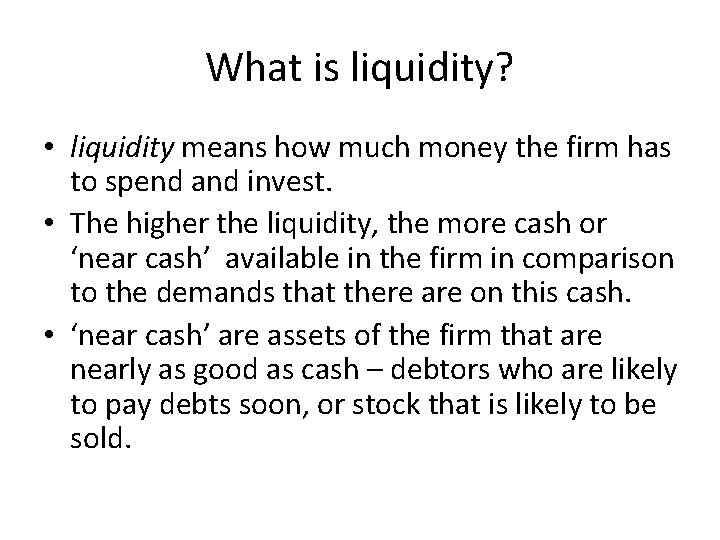What is liquidity? • liquidity means how much money the firm has to spend and invest. • The higher the liquidity, the more cash or ‘near cash’ available in the firm in comparison to the demands that there are on this cash. • ‘near cash’ are assets of the firm that are nearly as good as cash – debtors who are likely to pay debts soon, or stock that is likely to be sold.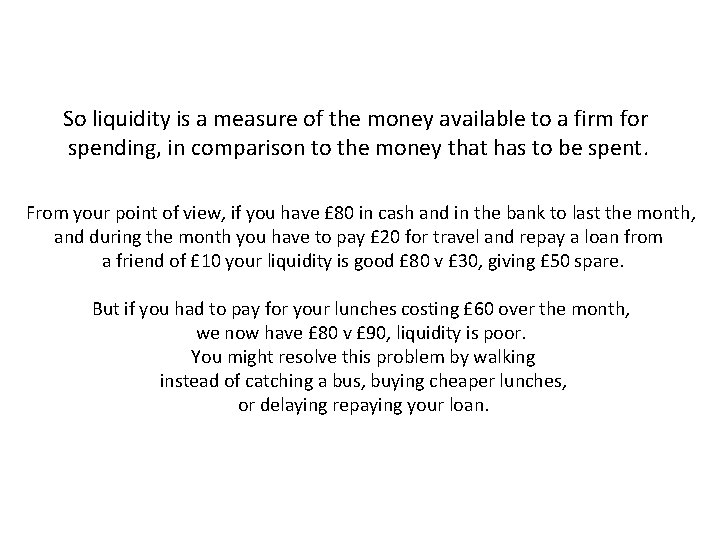So liquidity is a measure of the money available to a firm for spending, in comparison to the money that has to be spent. From your point of view, if you have £ 80 in cash and in the bank to last the month, and during the month you have to pay £ 20 for travel and repay a loan from a friend of £ 10 your liquidity is good £ 80 v £ 30, giving £ 50 spare. But if you had to pay for your lunches costing £ 60 over the month, we now have £ 80 v £ 90, liquidity is poor. You might resolve this problem by walking instead of catching a bus, buying cheaper lunches, or delaying repaying your loan.Working Capital is a measure of liquidity. If working capital is high, then liquidity is good. If working capital is low or even negative, then liquidity is poor. Using the examples of money v spending on the last slide. £ 80 in cash and in the bank to last the month, during the month £ 20 for travel and repay a loan from a friend of £ 10 your liquidity is good £ 80 v £ 30, giving £ 50 spare = £ 50 of working capital £ 80 in cash and in the bank to last the month, during the month £ 20 for travel and repay a loan from a friend of £ 10 plus your lunches costing £ 60 over the month, we now have £ 80 v £ 90, £ 10 short of what is needed = -£ 10 of working capital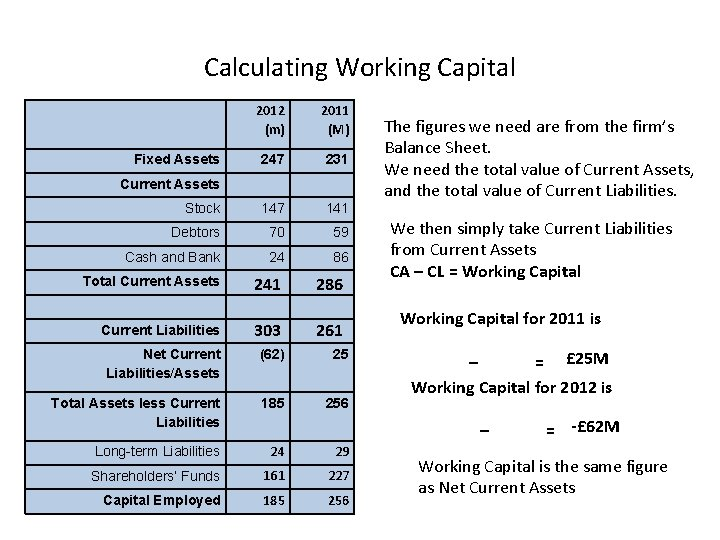Calculating Working Capital 2012 (m) 2011 (M) 247 231 Stock 147 141 Debtors 70 59 Cash and Bank 24 86 Total Current Assets 241 286 Current Liabilities 303 261 Net Current Liabilities/Assets (62) 25 Total Assets less Current Liabilities 185 256 Long-term Liabilities 24 29 Shareholders’ Funds 161 227 Capital Employed 185 256 Fixed Assets Current Assets The figures we need are from the firm’s Balance Sheet. We need the total value of Current Assets, and the total value of Current Liabilities. We then simply take Current Liabilities from Current Assets CA – CL = Working Capital for 2011 is – = £ 25 M Working Capital for 2012 is – = -£ 62 M Working Capital is the same figure as Net Current Assets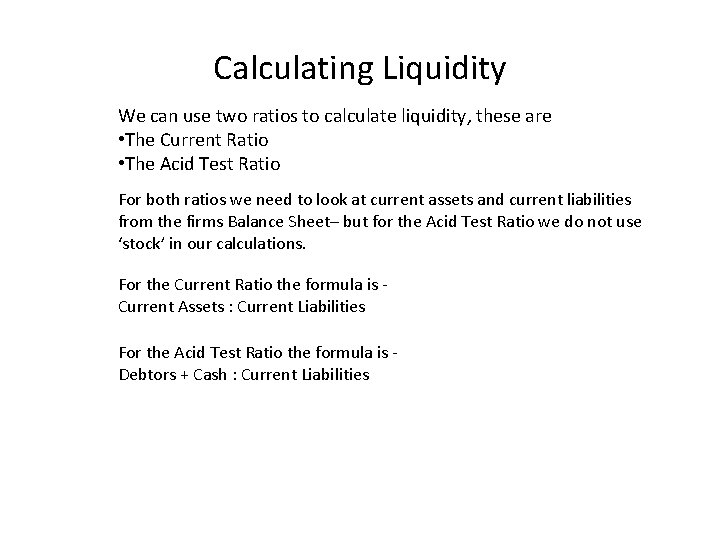Calculating Liquidity We can use two ratios to calculate liquidity, these are • The Current Ratio • The Acid Test Ratio For both ratios we need to look at current assets and current liabilities from the firms Balance Sheet– but for the Acid Test Ratio we do not use ‘stock’ in our calculations. For the Current Ratio the formula is Current Assets : Current Liabilities For the Acid Test Ratio the formula is Debtors + Cash : Current Liabilities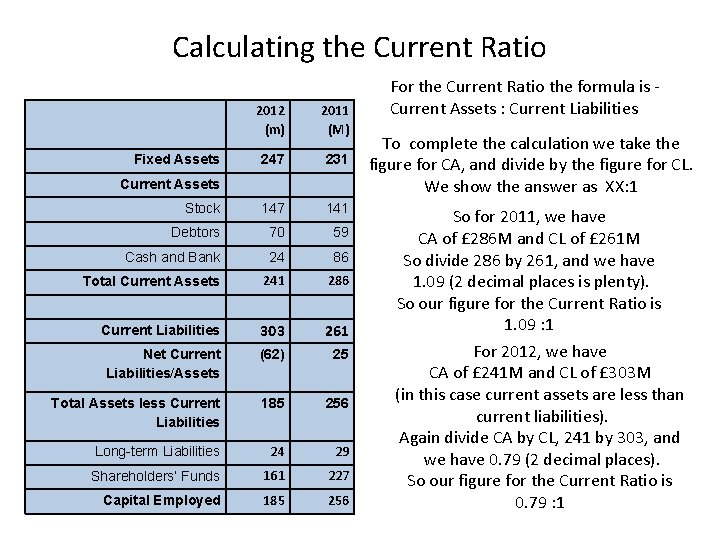Calculating the Current Ratio 2012 (m) 2011 (M) 247 231 Stock 147 141 Debtors 70 59 Cash and Bank 24 86 Total Current Assets 241 286 Current Liabilities 303 261 Net Current Liabilities/Assets (62) 25 Total Assets less Current Liabilities 185 256 Long-term Liabilities 24 29 Shareholders’ Funds 161 227 Capital Employed 185 256 Fixed Assets Current Assets For the Current Ratio the formula is Current Assets : Current Liabilities To complete the calculation we take the figure for CA, and divide by the figure for CL. We show the answer as XX: 1 So for 2011, we have CA of £ 286 M and CL of £ 261 M So divide 286 by 261, and we have 1. 09 (2 decimal places is plenty). So our figure for the Current Ratio is 1. 09 : 1 For 2012, we have CA of £ 241 M and CL of £ 303 M (in this case current assets are less than current liabilities). Again divide CA by CL, 241 by 303, and we have 0. 79 (2 decimal places). So our figure for the Current Ratio is 0. 79 : 1Commenting on the Current Ratiowhat do the figures we have calculated tell us? For 2011, we have a Current Ratio of 1. 09 : 1 For 2012, we have a Current Ratio of 0. 79 : 1 First of all remember we are measuring ‘liquidity’ - how much money the firm has to spend and invest. To have a good level of liquidity the firm should have more Current Assets than Current Liabilities. This would be shown by the ratio being more than 1: 1. So the Current Ratio could be 1. 2: 1, 1. 5: 1, or as much as 4: 1. The figures for the Current Ratio are 1. 09: 1, and 0. 79: 1. These indicate, a low and worsening level of liquidity. The firm may have problems meeting demands for payments from creditors and others (the bank if they have an overdraft). They are short of Working Capital. But what is an acceptable Current Ratio? What should a firm be aiming for?An acceptable Current Ratio - a good level of liquidity, or working capital As a guide to liquidity, if the Current Ratio figure is: • above 1. 5: 1, we can say that liquidity is good • between 1. 5: 1 and 1: 1, we can say it is reasonable • below 1: 1, we can say it is poor But do not treat the above figures as a fixed rule. Some firms happily survive on low current ratios. These typically are businesses with a high level of stock turnover, who deal in cash. Good examples of this type of firm are supermarkets. Tesco quite happily survives on a current ratio of about 0. 4: 1Calculating the Acid Test Ratio 2012 (m) 2011 (M) 247 231 Stock 147 141 Debtors 70 59 Cash and Bank 24 86 Total Current Assets 241 286 Current Liabilities 303 261 Net Current Liabilities/Assets (62) 25 Total Assets less Current Liabilities 185 256 Long-term Liabilities 24 29 Shareholders’ Funds 161 227 Capital Employed 185 256 Fixed Assets Current Assets For the Acid Test Ratio the formula is Debtors + Cash : Current Liabilities So we add together the figures for Debtors and Cash (at bank), and divide by the figure for CL. We show the answer as XX: 1 So for 2011 the figures are; £ 59 m + £ 86 m : £ 261 m = Acid Test Ratio of 0. 55 : 1 And for 2012 the figures are; £ 70 m + £ 24 m : £ 303 m = Acid Test Ratio of 0. 31 : 1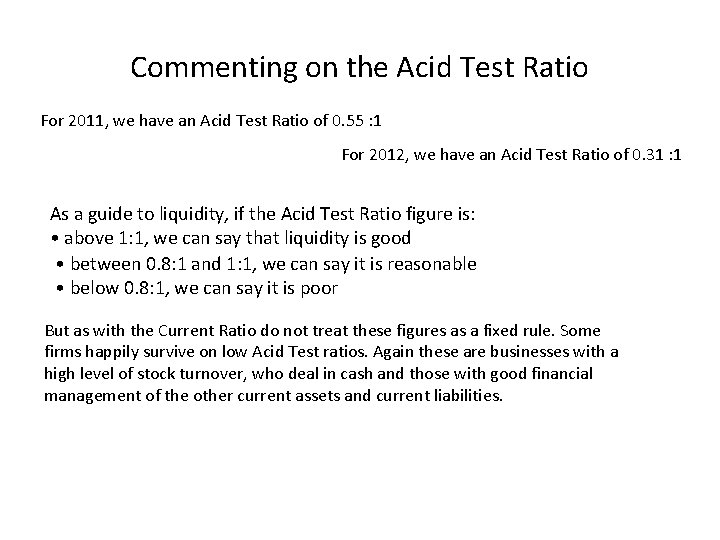Commenting on the Acid Test Ratio For 2011, we have an Acid Test Ratio of 0. 55 : 1 For 2012, we have an Acid Test Ratio of 0. 31 : 1 As a guide to liquidity, if the Acid Test Ratio figure is: • above 1: 1, we can say that liquidity is good • between 0. 8: 1 and 1: 1, we can say it is reasonable • below 0. 8: 1, we can say it is poor But as with the Current Ratio do not treat these figures as a fixed rule. Some firms happily survive on low Acid Test ratios. Again these are businesses with a high level of stock turnover, who deal in cash and those with good financial management of the other current assets and current liabilities.Commenting on the Acid Test Ratio If we look at the Acid Test Ratio, we see a figure of 0. 55: 1 for 2011, this tells us that liquidity is poor, and the business may have problems if creditors demanded the money they are owed. The working capital situation gets worse over the year, with the ratio falling to 0. 31: 1, the firm has nowhere near enough current assets to meet potential demands from creditors.Calculate the firms Current Ratios and Acid Test Ratios for both years. Comment on your findings. So for 2009 the figures are; Current Ratio 2543 : 1379 = 1. 84 : 1 Acid Test Ratio 2450 : 1379 = 1. 77 : 1 Was it worth calculating the ATR? So for 2010 the figures are; Current Ratio 3063 : 1549 = 1. 97 : 1 Acid Test Ratio 2980 : 1549 = 1. 92 : 1 Was it worth calculating the ATR?